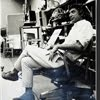## Eugene Ray SDSU 1970'sEugene Ray 1970's. photo credit: Tom Davis

## Thursday, June 26, 2014

### FOR DAVE FOBES / my admiration & gratitude

+"THE RED SEE SCROLLS"+
>one night showing of dave fobes<
>recent work is saturday evening<
+A MUST SEE EVENT+ !!!
>and it shall be grand for us all< !
^^^^^^^^^^^^^^^^^^^
+I AM REMINDED OF SO MANY+
+DAVE FOBES, ARTIST+
>(all of the first rank)< !!!
+FRIEND, SDSU-ED ALUM+
+AND DEVOTED PROF+
^^^^^^^^^^^^^^
>tonights blog is my homage to<
+DEEP GRATITUDE+
>dave for these many reasons<
^^^^^^^^^^^^^^^^^^^^
>ralph bowman (left) and dave at the lunch<
>which galvanized the beginning of this<
+DAVE the DEVOTED+
>blog which dave administered a year<
^^^^^^^^^^^^^^^^^^^^^^^
>dave's craftsmanship, second to none is<
>seen here in my design for a yogurt<
+DAVE the CRAFTSMAN+
>shop for our friend larry urruttia<
^^^^^^^^^^^^^^^^^^^^
>i was very impressed when dave took my<
>recommendation and purchased this very<
+DAVE the SCHOLAR+
^^^^^^^^^^^^^^^^^^^^^^^^
>ufo and crop circle technology as seen here<
>are subjects i can freely discuss with dave<
+DAVE the UFOLOGIST+
>where with some it is very difficult data<
^^^^^^^^^^^^^^^^^^^^^^^^^
>chris dunn's transcendent research into<
>egyptian / extraterrestrial technology<
+DAVE the MACHINIST+
>is a subject dave is at home with<
^^^^^^^^^^^^^^^^^^^^^
>dave was the star of my classes in the<
>early years when we were looking for<
+DAVE the STUDENT+
>housing of a kit of parts affordability<
^^^^^^^^^^^^^^^^^^^^^^^^
>the famed et-egyptian djed device as seen<
>here w/ eric von danniken is something<
+DAVE the VISIONARY+
>i believe dave will master someday<
^^^^^^^^^^^^^^^^^^^^^^^
>this ancient cosmic-sumerian time piece,<
>centuries ahead of its time, is the type of<
+DAVE the INVENTOR+
>mechanism dave is capable to construct<
^^^^^^^^^^^^^^^^^^^^^^^^^
>dave's aid in helping me build the silver<
>ship was superb despite a very small<
+DAVE the BELIEVER+
>budget, difficult site & harsh winter<
^^^^^^^^^^^^^^^^^^^^^^^
>dave and jim bourke assembled the shelves<
>for my library that means so much to me<
+DAVE the PROFESSOR+
>in one fantastic day of craftsmanship<
^^^^^^^^^^^^^^^^^^^^^^^^
>dave's fabulous paintings of his cosmic<
>series won my heart w/ its kinship to<
+DAVE the ARTIST+
>our shared vision for et biotronics<
^^^^^^^^^^^^^^^^^^^^^^>dave is an alumnus i feel who would be<
>happy in the company of leonardo in<
+DAVE the FUTURIST+
>his workshop exploring the future<
^^^^^^^^^^^^^^^^^^^^^^^
>dave shall live long enough to explore<
>wormhole time travel evolution as he<
+DAVE the EXPLORER+
>did while in my synergetics class<
^^^^^^^^^^^^^^^^^^^^^
+WHEN I LEAVE THIS PLANET+
>(please see "the red sea scrolls"<
>1955 julian ave, san diego<
>sat., june 28th, 5-8 pm)<
+MY SHOP IS IN GOOD CARE+ !
^^^^^^^^^^^^^^^^^^^^^^
eugene ray, mfa, architect
professor emeritus, sdsu